# LOGNORM.DIST Function

Calculates the cumulative log-normal distribution function at a given value of x

## Lognormal Distribution Excel Function

The Lognormal Distribution Excel Function is categorized under Excel Statistical functions. It will calculate the cumulative lognormal distribution function at a given value of x. We can use the function to analyze data that‘s been logarithmically transformed.

A variable x is log-normally distributed if its natural logarithm is normally distributed.

In terms of Excel functions: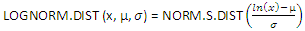Where μ is the mean of ln(x) and σ is the standard deviation of ln(x).

In financial analysis, the LOGNORM.DIST function is often used in analyzing stock prices, as normal distribution cannot be used to model stock prices. The normal distribution includes a negative side, but stock prices cannot fall below zero.

Also, the function is useful in pricing options. The Black-Scholes model uses the lognormal distribution as its basis to determine option prices.

### Formula

=LOGNORM.DIST(x,mean,standard_dev,cumulative)

The LOGNORM.DIST function uses the following arguments:

1. X (required argument) – This is the value at which we wish to evaluate the function.
2. Mean (required argument) – The mean of In(x).
3. Standard_dev (required argument) – This is the standard deviation of In(x).
4. Cumulative (optional argument) – This specifies the type of distribution to be used. It can be either TRUE (implies the cumulative distribution function) or FALSE (implies the normal probability density function).

### How to use the Lognormal Distribution Excel Function

To understand the uses of the LOGNORM.DIST function, let’s consider an example:

#### Lognormal Distribution Example

Suppose we are given the following data:

###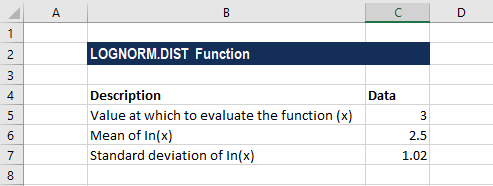The formula for calculating cumulative lognormal distribution is shown below: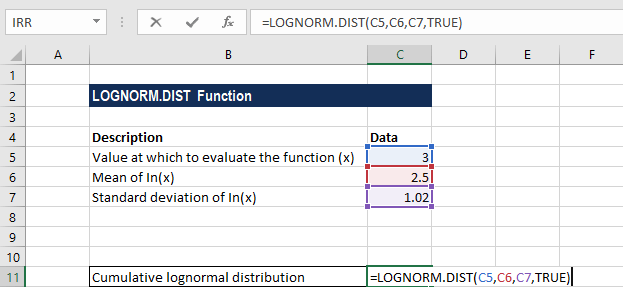We get the result below: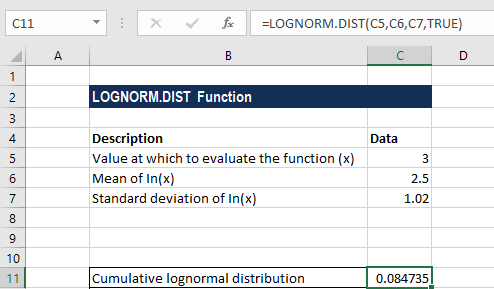The formula for calculating probability lognormal distribution is shown below: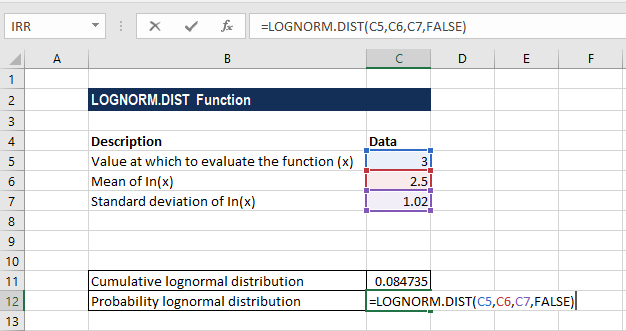We get the result below: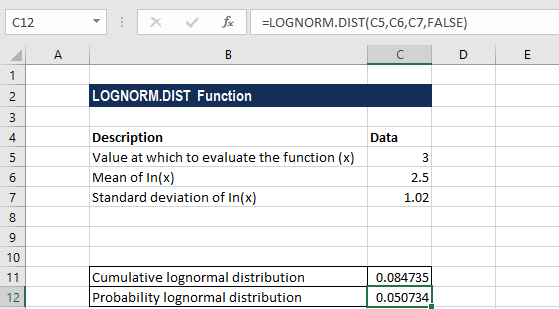### Notes about the Excel Lognormal Distribution Function

1. The LOGNORM.DIST function will truncate all numerical values to integers.
2. #NUM! error – Occurs when:
• The argument x given is less than or equal to zero; or
• The argument standard_dev is less than or equal to zero.
3. #VALUE! error – Occurs when any of given arguments is non-numeric.
4. The LOGNORMAL.DIST function was introduced in Excel 2010 and hence is unavailable in earlier versions. For older versions, we can use the LOGNORMDIST function.

### Free Excel Course

Check our Free Excel Crash Course to learn more about Excel functions using your own personal instructor. Master Excel functions to create more sophisticated financial analysis and modeling toward building a successful career as a financial analyst.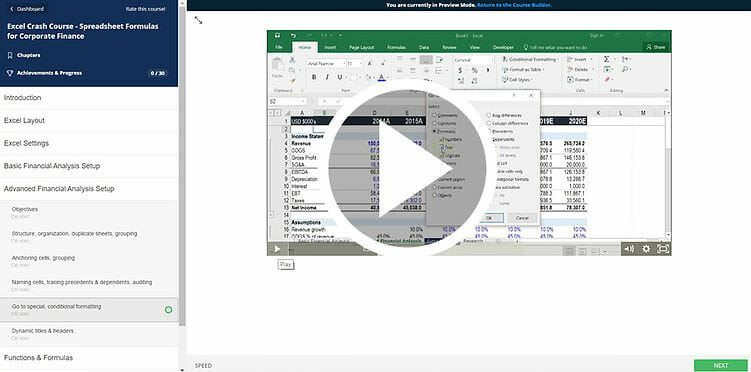Thanks for reading CFI’s guide to important Excel functions! By taking the time to learn and master these functions, you’ll significantly speed up your financial modeling. To learn more, check out these additional CFI resources:

• Excel Formulas for Finance